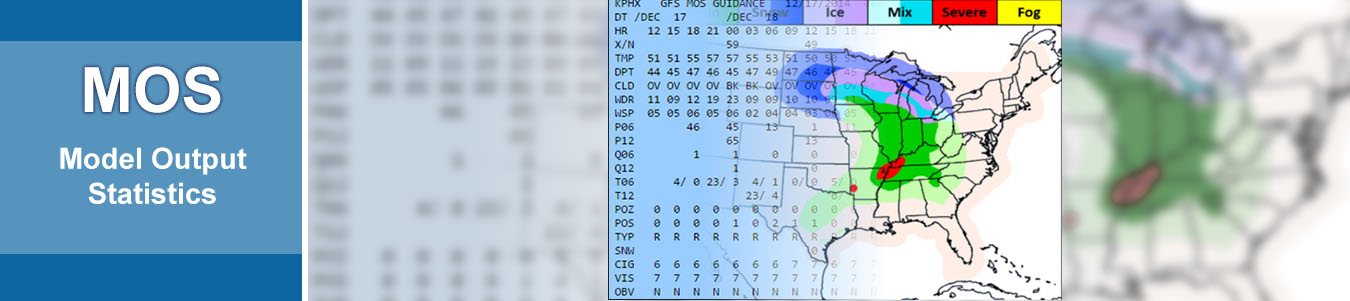## What is MOS?

Model Output Statistics (MOS) is a type of statistical post-processing, a class of techniques used to improve numerical weather models' ability to forecast by relating model outputs to observational or additional model data. MOS was defined by Glahn and Lowry (pdf) in 1972 as the following:

Model Output Statistics is an objective weather forecasting technique which consists of determining a statistical relationship between a predictand and variables forecast by a numerical model at some projection time(s). It is, in effect, the determination of the "weather related" statistics of a numerical model.

The predictors MDL currently uses are:

• NWP Model Forecasts
• Prior Observations
• Geoclimatic Data

Predictor points can come from either point observations or from data that has been calculated on / interpolated to points on a grid. The MOS products which are more heavily based on true observation points are referred to as station-based mos, while MOS generated based on gridded data is called gridded MOS (GMOS).

The models used for MOS data also can be changed. MDL is currently producing MOS based on the GFS, Ensemble GFS, and NAM models.

The statistical method used by MDL is multiple linear regression (with forward selection). Other techniques are possible, such as: Polynomial or logistic regression; or neural networks. (For more information on these and other statistical techniques, see: Wilks, 2006: Statistical Methods in the Atmospheric Sciences).

# Why MOS?  Advantages & Limitations

## What MOS Does

• Objectively interprets NWP Model based on historical sample
• Predicts events forced by synoptic-scale systems
• Corrects for certain systematic NWP model biases
• Mimics conceptual forecast models
• Quantifies uncertainty in NWP model forecasts
• Accounts for deterioration NWP model skill with increasing forecast projection
• Accounts for some local effects
• Incorporates climatic considerations

## What MOS Does NOT Do

• Account for forecaster excitement factor
• Predict events forced by mesoscale features
• Correct for systematic NWP model errors related to map type or synoptic situation
• Correct for certain deficiencies in NWP model physics, analysis schemes, or parameterizations
• Account for changes to NWP model components
• Account for EVERY local effect
• Account for unusual climatic conditions

## Advantages of MOS

• Mathematically simple, yet powerful
• Non-linearity can be modeled by using NWP variables and transformations
• Probability forecasts possible from a single run of NWP model
• Removal of some systematic model bias

## Limitations of MOS

• Need historical record of observations at forecast points (quality matters!)
• Short-term sample periods cannot be used
• Changing NWP models means re-configuring equations - with a new sample set

## Contact MOS

For further information about the current state of MDL's MOS initiatives, please contact Mark.Antolik@noaa.gov.